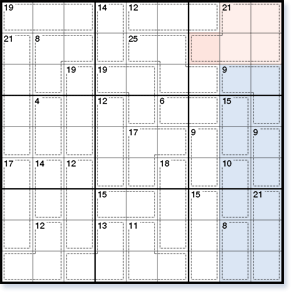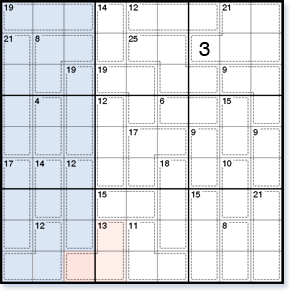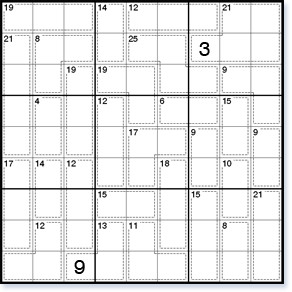# Killer Sudoku solving techniques

Killer Sudoku adds a new dimension to standard Sudoku, requiring arithmetic to solve. You will need specialised Killer Sudoku solving techniques to progress in these puzzles besides the standard Sudoku techniques.

## Multiple 45 Rule

The multiple 45 rule is an extension of the 45 rule. It applies the 45 rule to more than one row, column or block, taking them together as a larger region.Figure 1

In the eighth and ninth columns (highlighted in Fig. 1), all cages lie completely inside, with the exception of the 21 cage, so the multiple 45 rule can be used. The total of all numbers in two columns is always 45 × 2 = 90. The outside total, the total of all cages in these two columns including the 21 cage which sticks out, is 93. So, the outside square in the seventh column must be 93 – 90 = 3.

This Killer Sudoku contains another example of the multiple 45 rule, using three columns.Figure 2

In the first, second and third columns (highlighted in Fig. 2), all cages lie completely inside, with the exception of the 13 cage. The total of all squares in three columns is 45 × 3 = 135. The inside total for these three columns, the total of all cages except the cage sticking out, is 126. So, the inside square in the third column is 135 – 126 = 9.Figure 3

In general, look for multiple rows, columns or blocks next to each other where all cages except one lie completely inside.